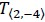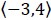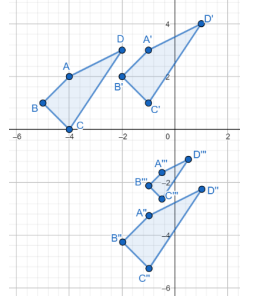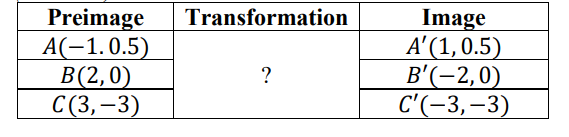# MA.912.GR.2.1Export Print
Given a preimage and image, describe the transformation and represent the transformation algebraically using coordinates.

### Examples

Example: Given a triangle whose vertices have the coordinates (-3,4), (2,1.7) and (-0.4,-3). If this triangle is reflected across the y-axis the transformation can be described using coordinates as (x,y)→(-x,y) resulting in the image whose vertices have the coordinates (3,4), (-2,1.7) and (0.4,-3).

### Clarifications

Clarification 1: Instruction includes the connection of transformations to functions that take points in the plane as inputs and give other points in the plane as outputs.

Clarification 2: Transformations include translations, dilations, rotations and reflections described using words or using coordinates.

Clarification 3: Within the Geometry course, rotations are limited to 90°, 180° and 270° counterclockwise or clockwise about the center of rotation, and the centers of rotations and dilations are limited to the origin or a point on the figure.

General Information
Subject Area: Mathematics (B.E.S.T.)
Strand: Geometric Reasoning
Status: State Board Approved

## Benchmark Instructional Guide

### Terms from the K-12 Glossary

• Coordinate Plane
• Dilation
• Origin
• Reflection
• Rigid Transformation
• Rotation
• Scale Factor
• Translation

### Vertical Alignment

Previous Benchmarks

Next Benchmarks

### Purpose and Instructional Strategies

In grade 8, students developed an understanding of single transformations. In Algebra 1, students extended this knowledge of transformations to transforming functions using tables, graphs and equations. In Geometry, students will understand transformations as functions and will transform figures, using two or more transformations, using words and coordinates. In later courses, types of transformations will be expanded to include stretches that can transform functions and conic sections and conversions between rectangular and polar coordinates.
• Instruction includes describing transformations using words and coordinates. Common transformations are provided below.
• Rotations can be described algebraically as the following:
90° counterclockwise about the origin ($x$, $y$) → (−$y$, $x$)
90° clockwise about the origin ($x$, $y$) → ($y$, −$x$)
180° counterclockwise about the origin ($x$, $y$) → (−$x$, −$y$)
180° clockwise about the origin ($x$, $y$) → (−$x$, −$y$)
270° counterclockwise about the origin ($x$, $y$) → ($y$, −$x$)
270° clockwise about the origin ($x$, $y$) → (−$y$, $x$)
• Reflections can be described algebraically as the following:
Over the $x$-axis ($x$, $y$) → ($x$, −$y$)
Over the $y$-axis ($x$, $y$) → (−$x$, $y$)
Over the line $y$$x$ ($x$, $y$) → ($y$, $x$)
Over the line $y$$x$ ($x$, $y$) → (−$y$, −$x$)
• Dilations
Dilation by a factor of a, where a is a real number ($x$, $y$) → ($a$$x$, $a$$y$)
• Translations
• Horizontal translation by $h$ units, where $h$ is a real number ($x$, $y$) → ($x$ + $y$, $y$) Vertical translation by $k$ units, where $k$ is a real number ($x$, $y$) → ($x$, $y$ + $k$) Horizontal translation by $h$ units, where $h$ is a real number, and vertical translation by $k$ units, where $k$ is a real number ($x$, $y$
• Instruction includes examining the effect of transforming coordinates by adding, subtracting, or multiplying the $x$- and $y$-coordinates with real-number values to make the connection between functions and transformations.
• For example, ΔPQR, with vertices P(−1, 4), Q(3, 4) and R(1, 7) can be transformed using the coordinates representation ($x$, $y$) → ($x$, $y$ − 1).
• For example, if the vertices of ΔABC are (4, −2), (4, 5) and (3, 3), respectively, and the vertices of ΔABC′ are (8, −4), (8, 10) and (6, 6), respectively, the coordinate representation can be determined.
• Instruction includes the use of hands-on manipulatives and geometric software for students to explore transformations.
• Instruction includes using a variety of ways to describe a transformation using coordinates. (MTR.2.1)
• For example, the same translation can be described using words as 2 units to the right and 4 units down, using coordinates as ($x$, $y$) → ($x$ + 2, $y$ − 4) or as $T$$x$,$y$ = ($x$ + 2, $y$ − 4), or.
• For example, a reflection over the $x$-axis can be represented as ($x$, $y$) → ($x$, −$y$) or as $r$$x$-axis ($x$, $y$) = ($x$, −$y$).
• For example, a 90°rotation counterclockwise about the origin can be represented as ($x$, $y$) → (−$y$, $x$) or as $R$0,90($x$, $y$) = (−$y$, $x$) where $O$ is the origin.
• The discussion of translations can be extended to include vectors when describing translations.
• For example, if a point is translated 3 units to the left and 4 units up the translation vector is. The vector summarizes the horizontal and vertical shifts.($x$ + $h$, $y$ + $k$)

### Common Misconceptions or Errors

• Students may believe the orientation of a figure would be conserved in a rotation in the same way that the orientation of a car, or gondola, is preserved when rotating on a Ferris wheel).
• Student may not be able to visualize some transformations like rotations. To address this, instruction includes using folding paper (e.g., patty paper) or interactive geometric software to allow students hands-on experiences and flexibility in exploration.

• Use the graph to the below to answer the following questions.• Part A. Describe the transformation that maps ABCD to A'B'C'D'
• Part B. Represent the transformation described in Part A algebraically.
• Part C. Algebraically represent the transformation needed to map A"B"C"D" onto ABCD
• Part D. Describe the transformation that maps A"B"C"D" onto A"'B"'C""D""
• Part E. How is the transformation described in Part D related to the transformation needed to map A"'B"'C""D"" onto A"B"C"D"• Part A. Ask students to plot A, B and C and A', B' and C' on the coordinate plane. What do you notice?
• Part B. How can you describe the transformation using words? Explore the patterns among the coordinates of the points of the preimages and the images.
• Part C. How can you describe the transformation using coordinate notation??

### Instructional Items

Instructional Item 1
• A triangle whose vertices are located at ($\frac{\text{2}}{\text{7}}$, −1), (−4,− $\frac{\text{14}}{\text{5}}$ ) and (3,1) is shifted to the right 2 units.
• Part A. What are the coordinates of the triangle after the translation?
• Part B. Describe the transformation that would map the preimage to the image algebraically.

*The strategies, tasks and items included in the B1G-M are examples and should not be considered comprehensive.

## Related Courses

This benchmark is part of these courses.
1200400: Foundational Skills in Mathematics 9-12 (Specifically in versions: 2014 - 2015, 2015 - 2022, 2022 and beyond (current))
1206310: Geometry (Specifically in versions: 2014 - 2015, 2015 - 2022, 2022 and beyond (current))
1206320: Geometry Honors (Specifically in versions: 2014 - 2015, 2015 - 2022, 2022 and beyond (current))
1206315: Geometry for Credit Recovery (Specifically in versions: 2014 - 2015, 2015 - 2022, 2022 and beyond (current))
7912065: Access Geometry (Specifically in versions: 2015 - 2022, 2022 and beyond (current))

## Related Access Points

Alternate version of this benchmark for students with significant cognitive disabilities.
MA.912.GR.2.AP.1a: Given a preimage and image, identify the transformation.
MA.912.GR.2.AP.1b: Select the algebraic coordinates that represent the transformation.

## Related Resources

Vetted resources educators can use to teach the concepts and skills in this benchmark.

## Formative Assessments

Transformations And Functions:

Students are given examples of three transformations and are asked if each is a function.

Type: Formative Assessment

Demonstrating Translations:

Students are asked to translate a quadrilateral according to a given vector.

Type: Formative Assessment

## MFAS Formative Assessments

Demonstrating Translations:

Students are asked to translate a quadrilateral according to a given vector.

Transformations And Functions:

Students are given examples of three transformations and are asked if each is a function.

## Student Resources

Vetted resources students can use to learn the concepts and skills in this benchmark.

## Parent Resources

Vetted resources caregivers can use to help students learn the concepts and skills in this benchmark.